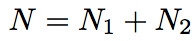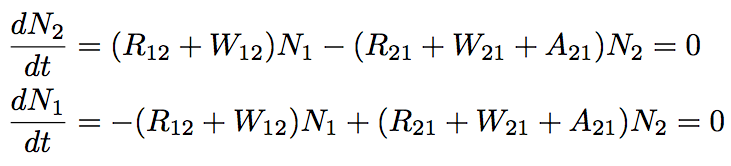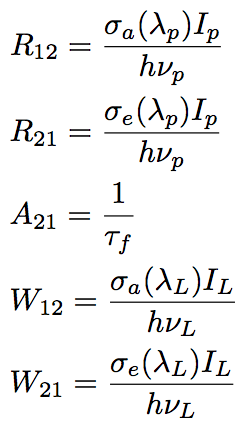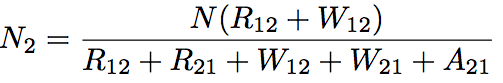A fundamental operation analysis will be shown by deriving simple rate equations from energy level of Yb silica glass. The sum of Yb3+ density in a ground and excited state (N1 and N2) equals the number of Yb3+ per unit volume (N).The rate equation of steady state is expressed as follows,where R12 and R21 are the pumping coefficients, W12 and W21 are the stimulated transition coefficients, and A21 is the spontaneous emission transition coefficient. These coefficients are obtained by follows,where λP, and λL are the wavelength of pumping, and signal light, respectively, τf is a lifetime of spontaneous emission (fluorescence lifetime of laser medium), and σa, and σe is the cross-section of absorption and spontaneous emission. These equations give N2 as follows.